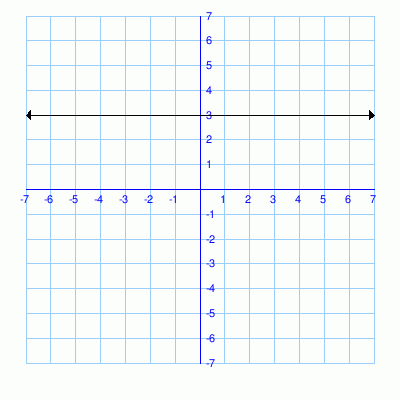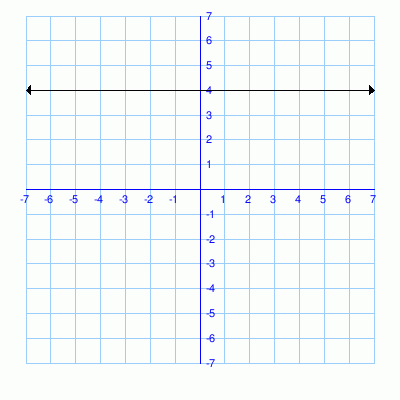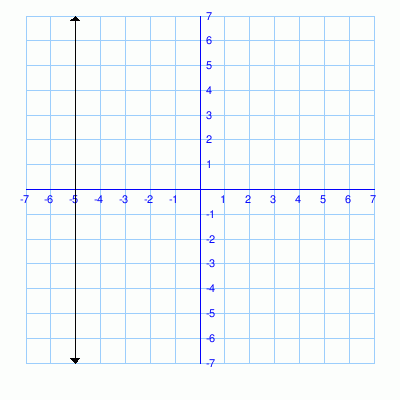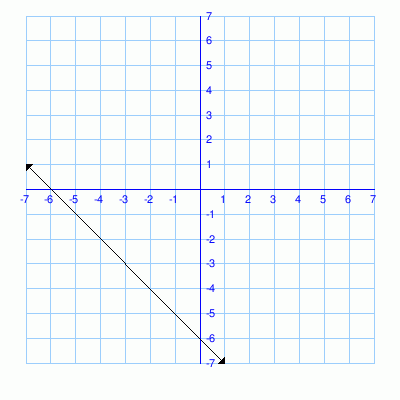# Math Practice Topic: Domain and Range

 Description: Determine the domain and range defined by - ordered pairs - graphs - function expressions, f(x) Adaptive Learning Progression: Ordered pairs, then graphs, then functions. Start using MathScore for free

## Sample Levels (out of 7)

Find the domain and range.
Use curly braces like {-2, 1, 5} for specific numbers, and parentheses like (-∞, ∞) for spans whose ends are exclusive.Use square brackets like [0, 5] for spans whose ends are inclusive.

 1 { (8, 13), (5, 12), (3, 2), (6, -1) } Domain: Range:

Find the domain and range.
Use curly braces like {-2, 1, 5} for specific numbers, and parentheses like (-∞, ∞) for spans whose ends are exclusive.Use square brackets like [0, 5] for spans whose ends are inclusive.

 1Domain: Range: 2Domain: Range: 3Domain: Range: 4Domain: Range:

Find the domain and range.
Use curly braces like {-2, 1, 5} for specific numbers, and parentheses like (-∞, ∞) for spans whose ends are exclusive.Use square brackets like [0, 5] for spans whose ends are inclusive.

1.
 f(x) = 3x+5
Domain:
2.
f(x) = √-x + 3 Domain: Range:
3.
f(x) = -3x2 + 1 Domain: Range:
4.
f(x) = √x - 5 Domain: Range: